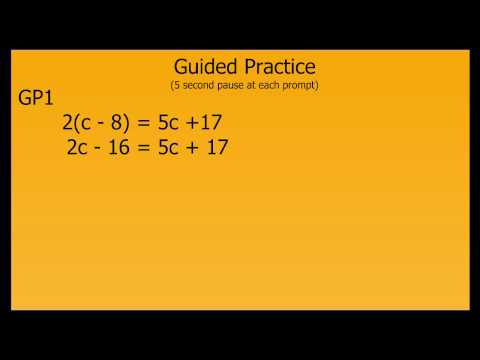### Solving Equations with Variables on Both Sides# Solving Equations with Variables on Both Sides

## 18 Video Interactions

1. 00:00:19

The goal is to...

• combine 4 - 2x to become 2x
• get the variables on one side of the equation and the constants on the other side of the equation

2. 00:00:44

True or false.

For this problem it is easier to subtract 5x from both sides as the first step.

• True.
• False.

3. 00:00:54

What's the next step?

• divide by 7
• add 17 to both sides.

4. 00:01:14

What inverse operation needs to be performed when given 21 = 7x?

• division.
• multiplication

5. 00:01:54

You may not know, but 1/8(24y - 48) can be efficiently simplified to 3y – 6 if you...

• recognize that when 1/8 is distributed out, both 24 and 48 will be divided by 8.

6. 00:02:05

Will the narrator choose to move y to the left or to the right side of the equation?

• Left side of the equation
• Right side of the equation.

7. 00:02:40

After substituting -6 for y, the narrator will follow the order of operations by simplifying the parentheses.

The narrator is choosing not to apply the distributive property--which is acceptable.

Why?

• There is less chance of error when applying the fraction to one number.

8. 00:04:39

What are we trying to do here?

• Solve the equation
• Isolate the variable to get c by itself

9. 00:04:52

What's the first step?

• move c to the left or right side of the equation.
• apply the distributive property.

10. 00:05:19

What should we do next?

• Subtract 2c from both sides.
• Subtract 5c from both sides.
• Add 16 to both sides.

11. 00:05:38

Now what?

• Subtract 17 from both sides.
• Add 16 to both sides.

12. 00:05:48

What inverse operation is next?

• Multiply 3 on both sides.
• Divide 3 on both sides.

13. 00:06:20

After -11 has been substituted, will the narrator simplify the parentheses or distribute 2 across -11 and 8?

• He will simplify the parentheses.
• He will distribute 2 across -11 and 8

14. 00:06:49

How do we simplify the right side of the equation?

• Apply the distributive property on the right side of the equation.
• Add 16w to both sides.

15. 00:06:57

Now what?

• Combine like terms on the right side of the equation
• Subtract 32 from both sides.
• Add 16w to both sides.

16. 00:07:18

Does this problem have no solution, one solution, or many solutions?

• No solution
• One solution
• Many solutions.

17. 00:08:36

What is the solution to this problem?

• 9 = n
• -19 = n

18. 00:09:07

What is the solution to this problem?

• 18 = 24 No solution.
• 18 = 18 Many solutions.
• 24 = 24 Many solutions.

Click here to preview, use, or make a copy of this interactive video lesson plan (bulb).